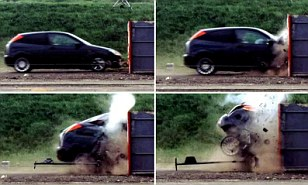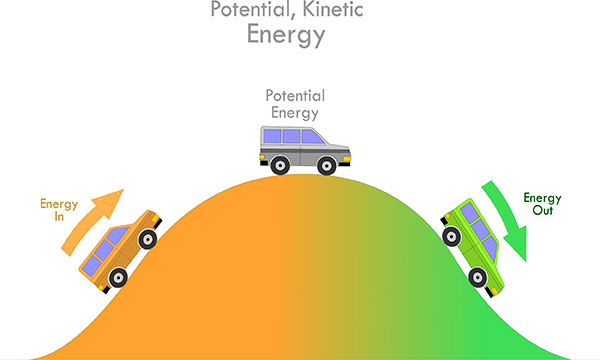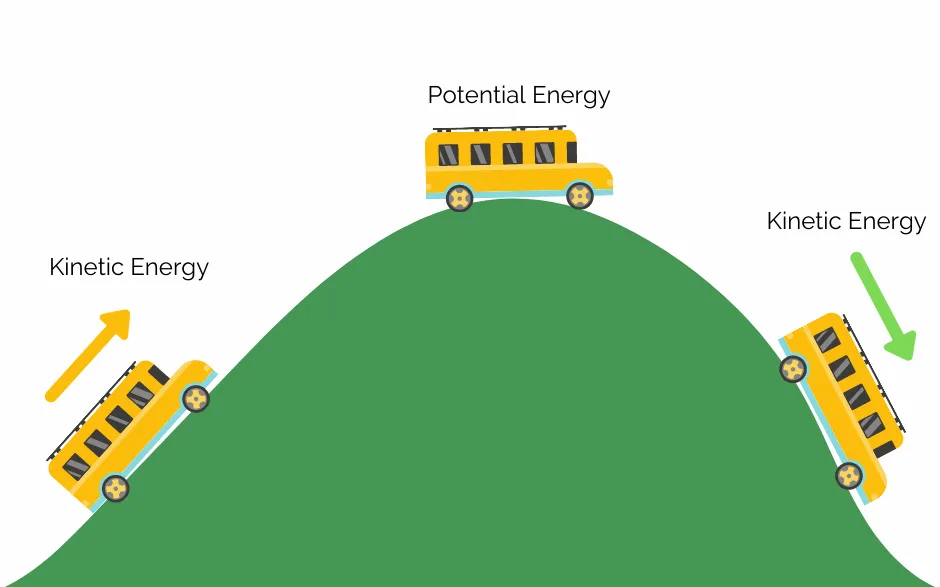Ravela Da Cruz

HS-PS2-3

# Kinetic Energy In Physics Study Guide

In this article, we learn about the definition of kinetic energy and its effects on physical objects.

## IntroductionSource

We’ve all seen collision videos of trains, cars, and bikes on the internet. The most obvious thing we see is that the faster the vehicle is going, the more damage it sustains. Two cars bumping into one another in slow-moving traffic is a fender bender at best. When a car that’s speeding at 100 miles an hour hits a solid wall, it gets completely wrecked. What’s the difference between the first and second scenarios? Lots of kinetic energy!

## ENERGY

• Energy is defined as the capacity to do work.
• It is measured in units called joule (J).
• Energy can exist in different forms in all objects, but the one which we commonly experience every day is Kinetic Energy.
• When we say “the capacity to do work,” it means anything from getting a stationary object to move to bringing moving objects to rest or changing the shape of the object.
• The energy in a system or an object can only be transformed from one form to another.

Energy can be used to perform work, or work can be done to transform energy from one form to another.

## KINETIC ENERGYSource

• Kinetic energy is the energy possessed by objects that are in motion.
• If a car is standing still on the top of a hill, it has zero kinetic energy (but lots of potential energy).
• When you give it a push and get it rolling over the slope, it begins to transform its potential energy into kinetic energy.

## THE FORMULA FOR KINETIC ENERGY

The formula for kinetic energy is given by:

KE = m

KE is the kinetic energy of the object

m is the mass of the moving object

v is the velocity of the moving object

The unit of kinetic energy is joules (J)

## DIFFERENCE BETWEEN KINETIC ENERGY AND POTENTIAL ENERGYSource

• Going back to the example of the car on top of a hill, we see that the vehicle has two types of energy.
• When you tow the car uphill, you are storing potential energy in the car by elevating its position and doing work against gravity.
• When it sits still on top of the hill, it is full of potential energy and zero kinetic energy.
• As the car gets rolling down the hill, the potential energy starts getting converted into kinetic energy.
• By the time the car is moving at the bottom of the hill, it is full of kinetic energy and zero potential energy.

# SUMMARY

• Energy is the capacity to do work. Energy and work are interchangeable.
• Kinetic energy is the energy possessed by moving objects.
• Kinetic energy can do work by changing the shape of objects (like in a car crash).
• Kinetic energy is directly proportional to the mass of the object and the square of its velocity.

## FAQs

Q. Is kinetic energy a vector or a scalar quantity?

Kinetic energy is a scalar quantity as it has only magnitude and no direction.

Q. Can the kinetic energy be negative?

By the definition of kinetic energy KE = m, we can see that mass cannot be negative, and the velocity also cannot be negative as the square of a negative number is positive.

Q. What happens to the kinetic energy when the speed decreases?

When speed decreases, the kinetic energy decreases as the square of the speed.

Q. When is the kinetic energy maximum?

Kinetic energy is maximum when objects reach the maximum possible velocity in their path of motion.

Q. How is the kinetic energy different from the potential energy?

Kinetic energy is the energy in moving objects, while potential energy is stored when the object is stationary.

We hope you enjoyed studying this lesson and learned something cool about Kinetic Energy! Join our Discord community to get any questions you may have answered and to engage with other students just like you! Don't forget to download our App to experience our fun VR classrooms - we promise, it makes studying much more fun!😎

1. Kinetic Energy: https://flexbooks.ck12.org/cbook/ck-12-physics-flexbook-2.0/section/6.6/primary/lesson/kinetic-energy-phys/ Accessed 11th April 2022
2. Energy: https://flexbooks.ck12.org/cbook/ck-12-middle-school-physical-science-flexbook-2.0/section/14.1/primary/lesson/energy-ms-ps/?referrer=crossref Accessed 11th April 2022
3. Kinetic Energy: https://byjus.com/physics/kinetic-energy/ Accessed 11th April 2022
4. What is Kinetic Energy? https://www.khanacademy.org/science/physics/work-and-energy/work-and-energy-tutorial/a/what-is-kinetic-energy Accessed 11th April 2022
5. Kinetic Energy: https://www.vedantu.com/physics/kinetic-energy Accessed 11th April 2022